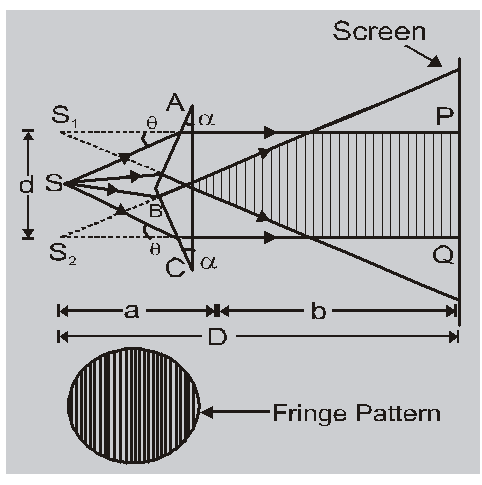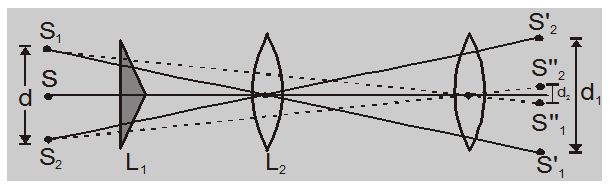Most Affordable JEE | NEET | 8,9,10 Preparation by Kota's Top IITian Doctor Faculties

# Fresnel biprism experiment - Physics - eSaral`

Hey, do you want to learn about the fresnel biprism experiment? If yes. Then keep reading.

## Fresnel's Biprism Experiment

1. It is an optical device to obtain two coherent sources by the refraction of lights.2. The angle of biprism is $179^{\circ}$ & refracting angle is $\alpha=1 / 2^{\circ}$.

3. Distance between source & screen D = a + b. Distance between two coherent sources

$=\mathrm{d}=2 \mathrm{a}(\mu-1) \alpha$

Where a = distance between source & Biprism

b = distance between screen & Biprism

$\mu$ = refractive index of the material of the prism.

$\lambda=\frac{d \beta}{D}=\frac{2 a(\mu-1) \alpha \beta}{(a+b)}$

$=\frac{\sqrt{d_{1} d_{2}} \cdot \beta}{(a+b)}$

Note-

$\alpha$ is in radian $\alpha^{0}=\alpha \times \frac{3.14}{180}$ Suppose refracting angle & refractive index is not known then d can be calculated by a convex lens.One convex lens whose focal length (f) and 4f < D. First convex lens is kept near biprism & $\mathrm{d}_{1}$ is calculated then it is kept near eyepiece & $\mathrm{d}_{2}$ is calculated. $\mathrm{d}=\sqrt{\mathrm{d}_{1} \mathrm{~d}_{2}}$

Application :

With the help of this experiment the wavelength of monochromatic light, the thickness of thin films, and their refractive index & distance between apparent coherent sources can be determined. When Fresnel's arrangement is immersed in water

(a) Effect on d

$\mathrm{d}_{\text {water }}<\mathrm{d}_{\mathrm{air}}$. Thus when the Fresnel's biprism experiment is immersed in water, then the separation between the two virtual sources decreases but in young's double-slit experiment it does not change.

(b) In young's double-slit experiment $\beta$ decreases and in Fresnel’s biprism experiment $\beta$ increases.

so, that's all from this article. I hope you get the idea about the fresnel biprism experiment. If you found this article informative then please share it with your friends. If you have any confusion related to this topic then feel free to ask in the comments section down below.

For a better understanding of this chapter, please check the detailed notes of the Wave Optics. To watch Free Learning Videos on physics by Saransh Gupta sir Install the eSaral App.